Complex Polynomial Functions (4): Degree n

We have already seen that the real polynomial functions of degree n can have, at most, n real zeros.We can consider the polynomial function that passes through a series of points of the plane. This is an interpolation problem that is solved here using the Lagrange interpolating polynomial.

When we consider a polynomial function of degree n in the complex plane it always have n roots. The Fundamental Theorem of Algebra states that every polynomial function of degree n has exactly n complex zeros, not necessarily distinct.

You start the applet seeing the representantion of a polynomial function of degree 5 whose zeros are the 5th roots of unity: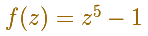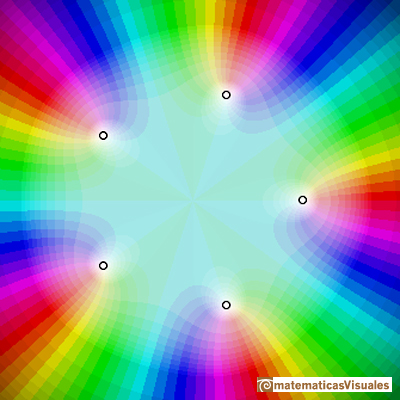You can change the position of the roots and then a more general polynomial function of degree 5 is represented.

You can change the degree of the polynomial and move the roots. These zeros can be repeated and then we say that they have a doble, triple and so on multiplicity.

The multiplicity of the zero is represented by the number of times that the color cycle (red->green->blue) goes round the root.

In this example you can see a reperesentatation of a polynomial function of degree 5 with a simple root and two double roots:This is a representation of a polynomial function of degree 6 with a simple root, a double root and a triple root: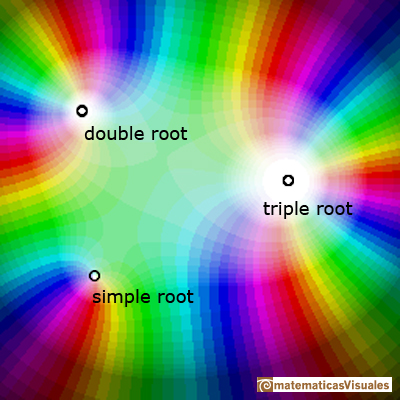You can see that the color cycle (red->green->blue) goes round the zero once, twice or three times.

You can see another video version with darker colors:

In the next video we play with a color code that uses a grid (or use the polar color code as before). You can see in black the points that are transformed into complex numbers with module "near" 1.

This is an example using the grid color code: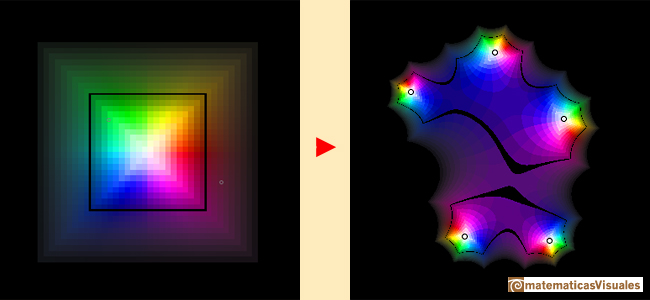The same polynomial function using a polar color code: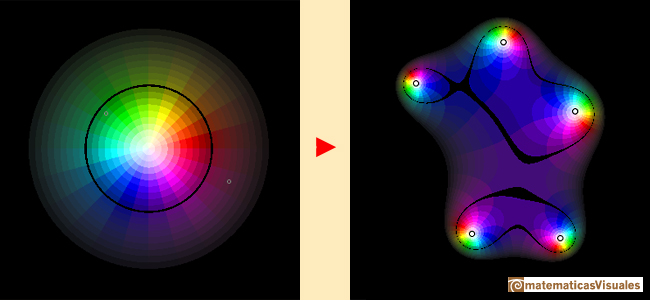REFERENCES

Tristan Needham - Visual Complex Analysis. Oxford University Press.Complex power functions with natural exponent have a zero (or root) of multiplicity n in the origin.A polynomial of degree 2 has two zeros or roots. In this representation you can see Cassini ovals and a lemniscate.Two points determine a stright line. As a function we call it a linear function. We can see the slope of a line and how we can get the equation of a line through two points. We study also the x-intercept and the y-intercept of a linear equation.Polynomials of degree 2 are quadratic functions. Their graphs are parabolas. To find the x-intercepts we have to solve a quadratic equation. The vertex of a parabola is a maximum of minimum of the function.Polynomials of degree 3 are cubic functions. A real cubic function always crosses the x-axis at least once.We can consider the polynomial function that passes through a series of points of the plane. This is an interpolation problem that is solved here using the Lagrange interpolating polynomial.Podemos modificar las multiplicidades del cero y del polo de estas funciones sencillas.Una primera aproximación a estas transformaciones. Representación de dos haces coaxiales de circunferencias ortogonales.The Complex Exponential Function extends the Real Exponential Function to the complex plane.The Complex Cosine Function extends the Real Cosine Function to the complex plane. It is a periodic function that shares several properties with his real ancestor.The Complex Cosine Function maps horizontal lines to confocal ellipses.Inversion is a plane transformation that transform straight lines and circles in straight lines and circles.Inversion preserves the magnitud of angles but the sense is reversed. Orthogonal circles are mapped into orthogonal circlesThe usual definition of a function is restrictive. We may broaden the definition of a function to allow f(z) to have many differente values for a single value of z. In this case f is called a many-valued function or a multifunction.Multifunctions can have more than one branch point. In this page we can see a two-valued multifunction with two branch points.The complex exponential function is periodic. His power series converges everywhere in the complex plane.The power series of the Cosine Function converges everywhere in the complex plane.We will see how Taylor polynomials approximate the function inside its circle of convergence.